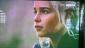# Differential Equation: Eliminate $C_1$, $C_2$, and $C_3$ from $y=C_1e^x+C_2e^{2x}+C_3e^{3x}$How to eliminate arbitrary constants
y=C1e^x+C2e^2x+C3e^3x

thank you :)### Plain text

• No HTML tags allowed.
• Lines and paragraphs break automatically.